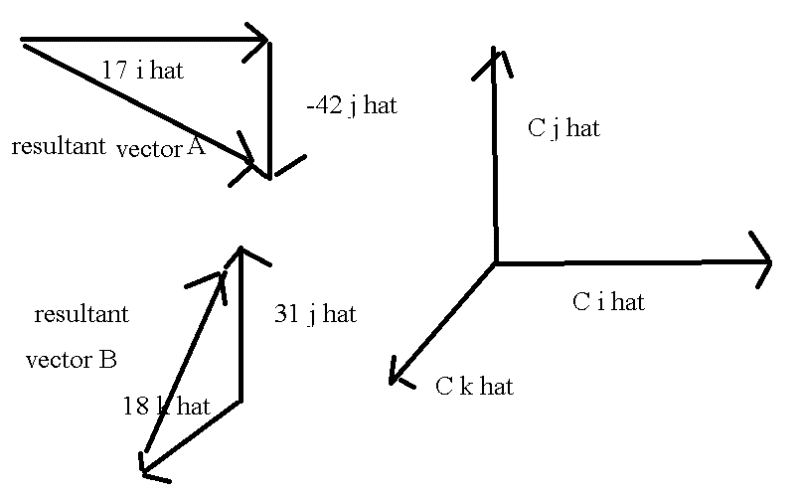# Solving components of vectors

## Homework Statement

Let resultant vector A = 17 i hat - 42 j hat and resultant vector B = 31 j hat + 18 k hat. Find the resultant vector C such that the sum of resultant vector A, B and C equals resultant vector zero. Find the i,j and k components of resultant vector C.

## Homework Equations

understand the basic of vectors i hat is in the x axis and j hat is the y axis. negative vector points down and left. positive vectors point right and up. use the tail to head rule to connect vectors.

## The Attempt at a SolutionI'm not sure how to go about solving this problem. Do I add the vectors with the same hats?